### Home > CALC > Chapter 9 > Lesson 9.4.1 > Problem9-114

9-114.
1. Consider the infinite series below. For each, decide if it converges or diverges and justify your conclusion. If the series converges, find its sum. Homework Help ✎

1.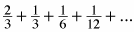2. 0.9 + 0.99 + 0.999 + 0.9999 + ...

3. 0.1 + 0.12 + 0.123 + 0.1234 + ...

4. 256 − 128 + 64 − 32 + ...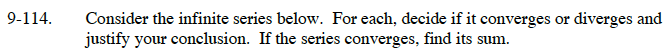For an infinite geometric series: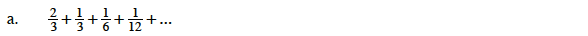This is a geometric series with r = 1/2.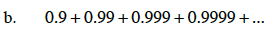Think of this as:
0.9 + (0.9 + 0.09) + (0.9 + 0.09 + 0.009) + ...
What is happening to the value of each term?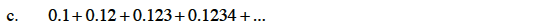See the hint in part (b).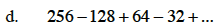This is a geometric series with r = –1/2.